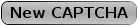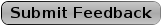Sudoku HowTo# X Wing and Other Fishy Things

The X Wing strategy looks for two rows that only have two instances of the same candidate in each row and the two instances of that candidate in each row are in the same two columns.

If this pattern is found then the number can be removed from any cells in the two columns that are not in the two rows where the initial candidates were found.

This process will also work when there is only two instances of a candidate in two columns and the two instances in the two columns are in the same two rows.

The other "Fishy Things" use the same concepts as X Wing. The Swordfish is based on three rows and three columns, the Jellyfish on four rows and four columns and the Squirmbag on five rows and five columns.
Figure 1 shows an X Wing pattern on the number 7 in rows 2 and 9. The number 7 is found in only the two blue cells in row 2 and only the two blue cells in row 9. The number 7 is located in the same columns in rows 2 and 9.

To place a number 7 in rows 2 and 9 the number 7 will have to be placed in diagonal corners of the square formed by the blue cells and it does not matter at this time which diagonal corners are used. Either of the two options places a number 7 in rows 2 and 9 and also places a number 7 in columns 4 and 9.

Any other instances of the number 7 in columns 4 and 9 can be removed.
Figure 2 shows an X Wing pattern on the number 7 and columns 2 and 6. The number 7 only exists in the blue cells in columns 2 and 6 and in both cases the number 7 is in rows 2 and 7.

The number 7 will have to be placed on the diagonal corners of the square created by the blue cells. Regardless of which diagonal corners the number 7 is placed in there will be a number 7 in columns 2 and 6 and there will also be a number 7 in rows 2 and 7.

The number 7 can be removed from all other cells in rows 2 and 7 that are not in columns 2 and 6.The Swordfish strategy looks for three rows that have instances of the considered candidate in each of the three rows and all instances of the considered candidate in these three rows are in the total of three columns.

If this pattern is found then the number can be removed from any cells in the three columns that are not in the three rows where the initial candidates were found.

This process will also work when there are instances of a considered candidate in three columns and these instances of the considered candidate in the three columns are all in a total of three rows.
Figure 3 shows a Swordfish pattern on the number 7 in rows 3, 5 and 8. The number 7 only exists in the blue cells in rows 3, 5 and 8 and in all cases the number 7 is in columns 2, 4 or 5 (Three total columns).

The number in question may be in all or only some of the three columns in each row for this pattern to work.

The number 7 will have to be placed in one of the blue cells in each of rows 3, 5 and 8 and because there is only three column possibilities in total there will be a number 7 in columns 2, 4 and 5 when this is done.

It does not matter which blue cells the 7s are placed in for rows 3, 5 and 8 at this time, but because there are three rows and only three possible columns, placing a 7 on each of the three rows will ensure there is a number 7 in columns 2, 4 and 5.

The number 7 can be removed from all other cells in columns 2, 4 and 5 that are not in rows 3, 5 and 8.
Figure 4 shows a Swordfish pattern on the number 3 in columns 1, 3 and 9. The number 3 only exists in the blue cells in columns 1, 3 and 9 and in all cases the number 3 is in rows 1, 5 or 9 (Three total rows).

The number in question may be in all or only some of the three rows in each column for this pattern to work.

The number 3 will have to be placed in one of the blue cells in each of columns 1, 3 and 9 and because there is only three row possibilities in total there will be a number 3 in rows 1, 5 and 9 when this is done.

It does not matter which blue cells the 3s are placed in for columns 1, 3 and 9 at this time, but because there are three columns and only three possible rows, placing a 3 on each of the three columns will ensure there is a number 3 in rows 1, 5 and 9.

The number 3 can be removed from all other cells in rows 1, 5 and 9 that are not in columns 1, 3 and 9.The Jellyfish strategy looks for four rows that have instances of the considered candidate in each of the four rows and all instances of the considered candidate in these four rows are in the total of four columns.

If this pattern is found then the number can be removed from any cells in the four columns that are not in the four rows where the initial candidates were found.

This process will also work when there are instances of a considered candidate in four columns and these instances of the considered candidate in the four columns are all in a total of four rows.
Figure 5 shows a Jellyfish pattern on the number 7 in rows 1, 2, 4 and 8. The number 7 only exists in the blue cells in rows 1, 2, 4 and 8 and in all cases the number 7 is in columns 1, 3, 7 or 8 (Four total columns).

The number in question may be in all or only some of the four columns in each row for this pattern to work.

The number 7 will have to be placed in one of the blue cells in each of rows 1, 2, 4 and 8 and because there is only four column possibilities in total there will be a number 7 in columns 1, 3, 7 and 8 when this is done.

It does not matter which blue cells the 7s are placed in for rows 1, 2, 4 and 8 at this time, but because there are four rows and only four possible columns, placing a 7 on each of the four rows will ensure there is a number 7 in columns 1, 3, 7 and 8.

The number 7 can be removed from all other cells in columns 1, 3, 7 and 8 that are not in rows 1, 2, 4 and 8.

9 July 2014
by John
RE: Comments and feedback for fishyThings

Hi. Thanks for pointing out this problem. It has to do with the way I create the images for the examples. The 7 can be removed from cells 2,2 and 3,2 because of the naked set of 6 and 7 in cells 6,2 and 7,2 and then the Jelly Fish works. This is fixed in the example now.
4 November 2012
by andreas
RE: Comments and feedback for fishyThings

hi john
Figure 5 actually shows a Jellyfish but i think candidates given do not fit. cell 2,2 shows number 7 but is not marked blue as other 7's are in row 2.
regarding this jellyfish pattern would not match. but there is naked triple 167 in cells (row/col) 1/3,2/1,3/1. with 7 removed from cell 2,2 (and 67 from cell 3,2) the blue cells match the jellyfish pattern. am i right?

Responses to articles are public.

Use the contact option on the menu to send an email to the website administrator if you do not want your comment visible to all users.
* Feedback
* Enter the letters from the CAPTCHA image.* Required InputTo submit this form, enter the letters you see on the red CAPTCHA image in the box next to the image. If you cannot read the image click 'New CAPTCHA' to get a different CAPTCHA image.

Using CAPTCHA verification helps reduce SPAM and web site abuse by making it harder for other computers to automatically access pages on this website.Sudoku Rules and Techniques

Sudoku Rules

Acknowledgments

I would like to acknowledge these sites referenced for strategy ideas and solving techniques.

SudokuWiki.Org by Andrew Stuart
PaulsPages.co.uk by Paul Stevens